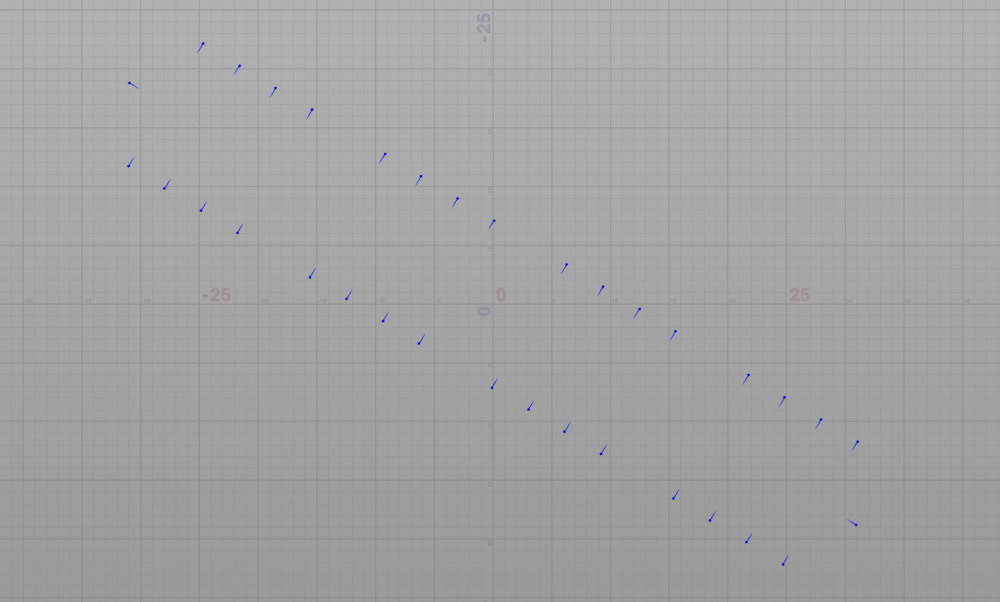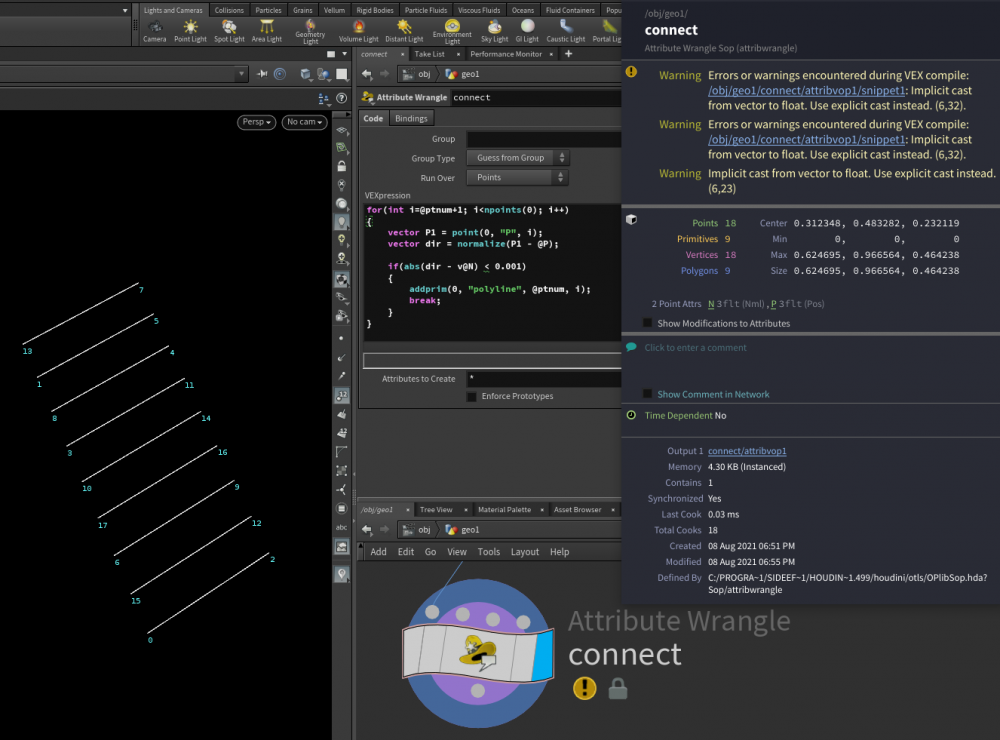## Recommended Posts

Hey hey. I'm trying to do a very simple thing - connect opposite points within vex... Normalas are given. I've tried with dot product but failed(((##### Share on other sites

Hello,

In a Point Wrangle, make a for loop going over every points, and compute the direction it has with the "current one".
Points that faces each other will match the Normal, and others won't.
So, if some point match with the normal of the current point, then you know you need to connect those points together.

As for the connecting, we can't really connect all points when they found a match, because you'd have two lines for each pair.
What I propose is to only make the connection when the match has an id higher than the current point.
So, say the match is 9 and 15.
9 matches with 15, 9 is lower than 15, so a line is created. 15 also match with 9, but 15 is higher than 9, so we don't connect.
(In the example below, I dodge this issue altogether by not including points with a lower id than the current point in the loop)

Here's untested code, to "visually" explain what I mean.
This should go in an Attribute Wrangle, running over Points.

```// We loop over the other points.
// We start at the current point +1,
// so we know we will never have "double-match"
for(int i=@ptnum+1, i<npoints(0); i++)
{
vector P1 = point(0, "P", i); // Get the position of the other point
vector dir = normalize(P1 - @P); // Get the direction of the other point relative to the current one

if(dir == v@N) // If the direction match with the normal
{
break; // Since we have a match, we know that we need to stop looking for other points.
}
}```

That's the idea anyways. Maybe the computed "dir" won't match perfectly with the Normal, and you'll need some threshold to compare dir and N (if equal within a margin of 0.001 or whatever).
A quick way to do that is abs( a - b ) <= threshold. (I think that should work for vectors, but it might not be the ideal way to do it. Although it should be fine for your case)

EDIT :
I quickly recreated the scene, and it seems to workI had to use the "almost equal" trick, because it had connected only 2 out of the 9 pairs.

Next time, please share a scene file.

connect_by_dir.hipnc

Edited by Alain2131
•1

##### Share on other sites

Removed my comment, @Alain2131's is more effective.

Edited by Fenolis
•1

##### Share on other sites
On 09/08/2021 at 12:58 AM, Alain2131 said:

Hello,

In a Point Wrangle, make a for loop going over every points, and compute the direction it has with the "current one".
Points that faces each other will match the Normal, and others won't.
So, if some point match with the normal of the current point, then you know you need to connect those points together.

As for the connecting, we can't really connect all points when they found a match, because you'd have two lines for each pair.
What I propose is to only make the connection when the match has an id higher than the current point.
So, say the match is 9 and 15.
9 matches with 15, 9 is lower than 15, so a line is created. 15 also match with 9, but 15 is higher than 9, so we don't connect.
(In the example below, I dodge this issue altogether by not including points with a lower id than the current point in the loop)

Here's untested code, to "visually" explain what I mean.
This should go in an Attribute Wrangle, running over Points.

```
// We loop over the other points.
// We start at the current point +1,
// so we know we will never have "double-match"
for(int i=@ptnum+1, i<npoints(0); i++)
{
vector P1 = point(0, "P", i); // Get the position of the other point
vector dir = normalize(P1 - @P); // Get the direction of the other point relative to the current one

if(dir == v@N) // If the direction match with the normal
{
break; // Since we have a match, we know that we need to stop looking for other points.
}
}```

That's the idea anyways. Maybe the computed "dir" won't match perfectly with the Normal, and you'll need some threshold to compare dir and N (if equal within a margin of 0.001 or whatever).
A quick way to do that is abs( a - b ) <= threshold. (I think that should work for vectors, but it might not be the ideal way to do it. Although it should be fine for your case)

EDIT :
I quickly recreated the scene, and it seems to workI had to use the "almost equal" trick, because it had connected only 2 out of the 9 pairs.

Next time, please share a scene file.

Thank you so much!!! It really helped me)) (Sorry for late answer)

## Create an account

Register a new account